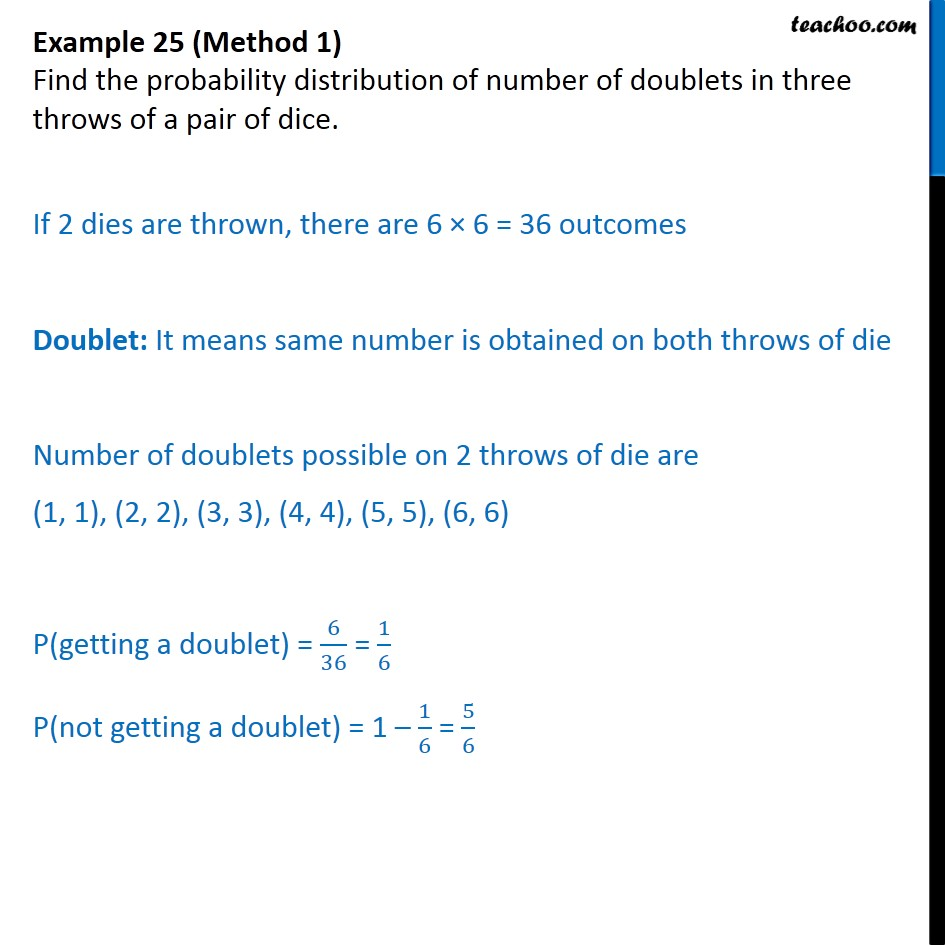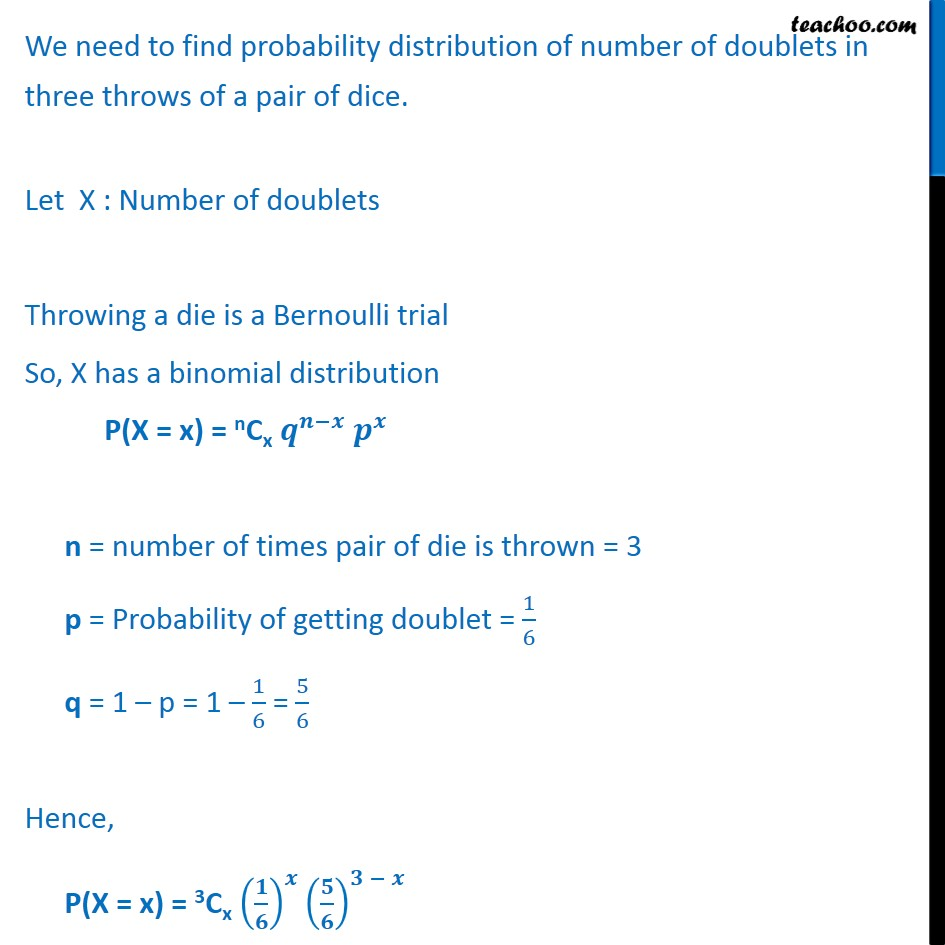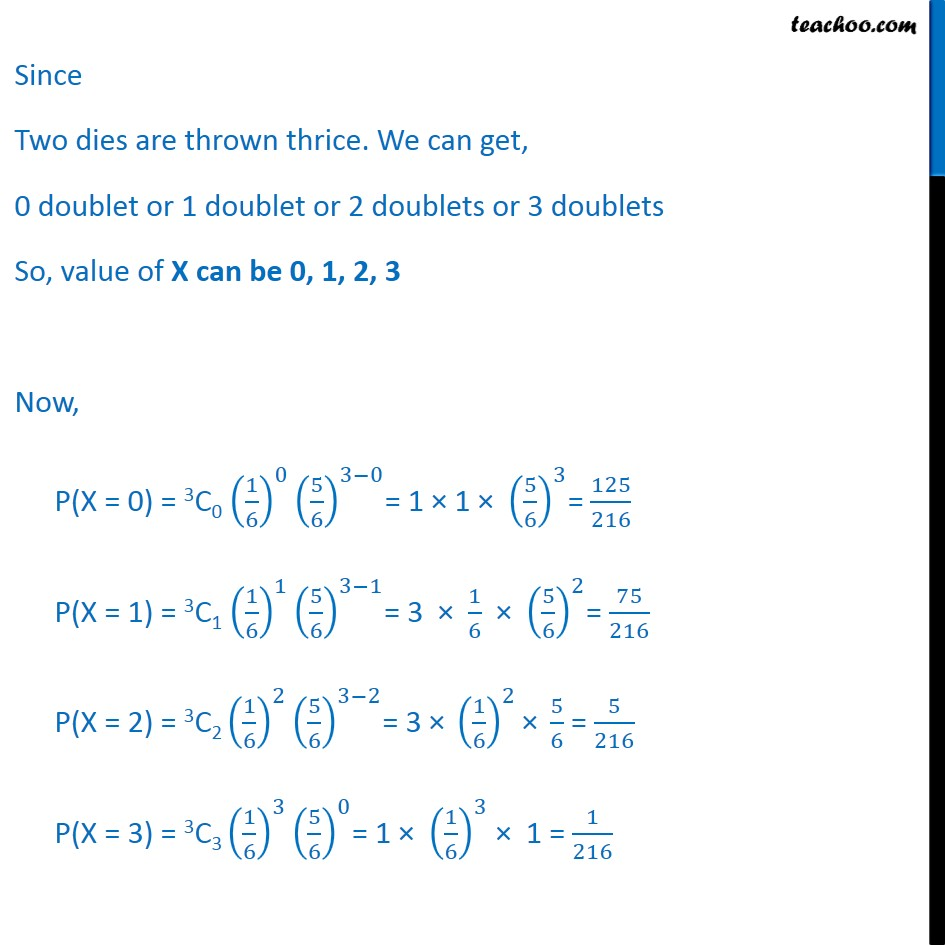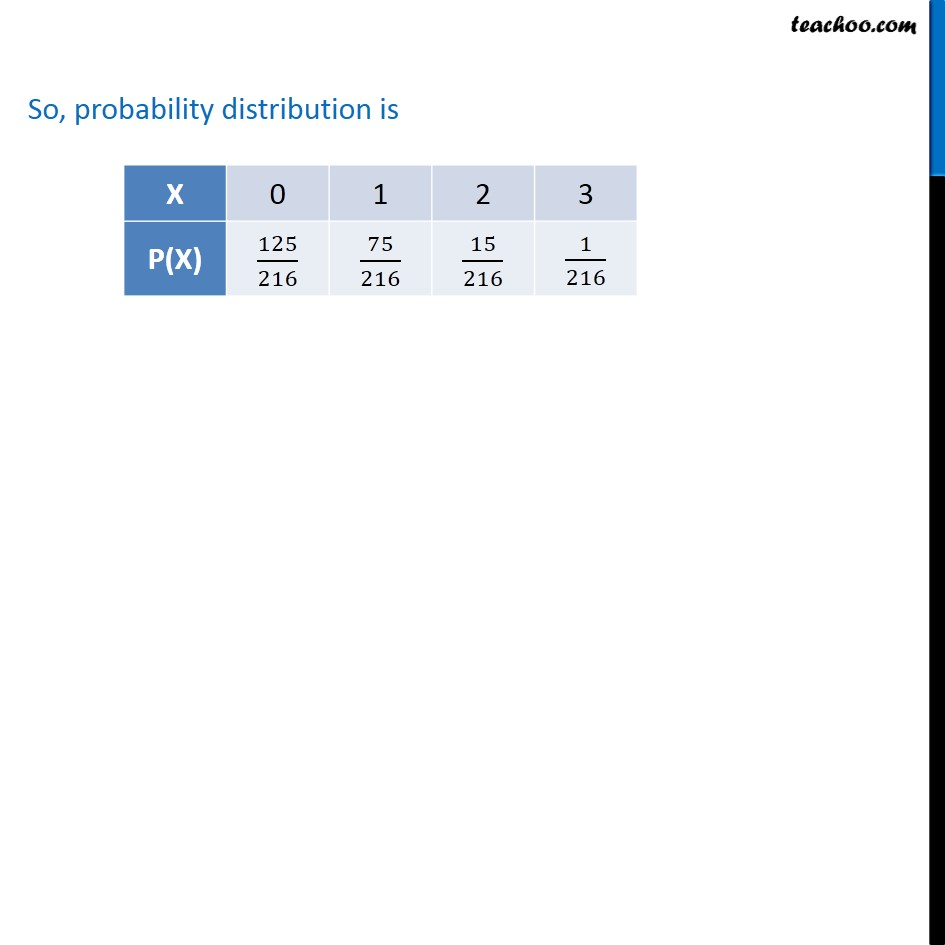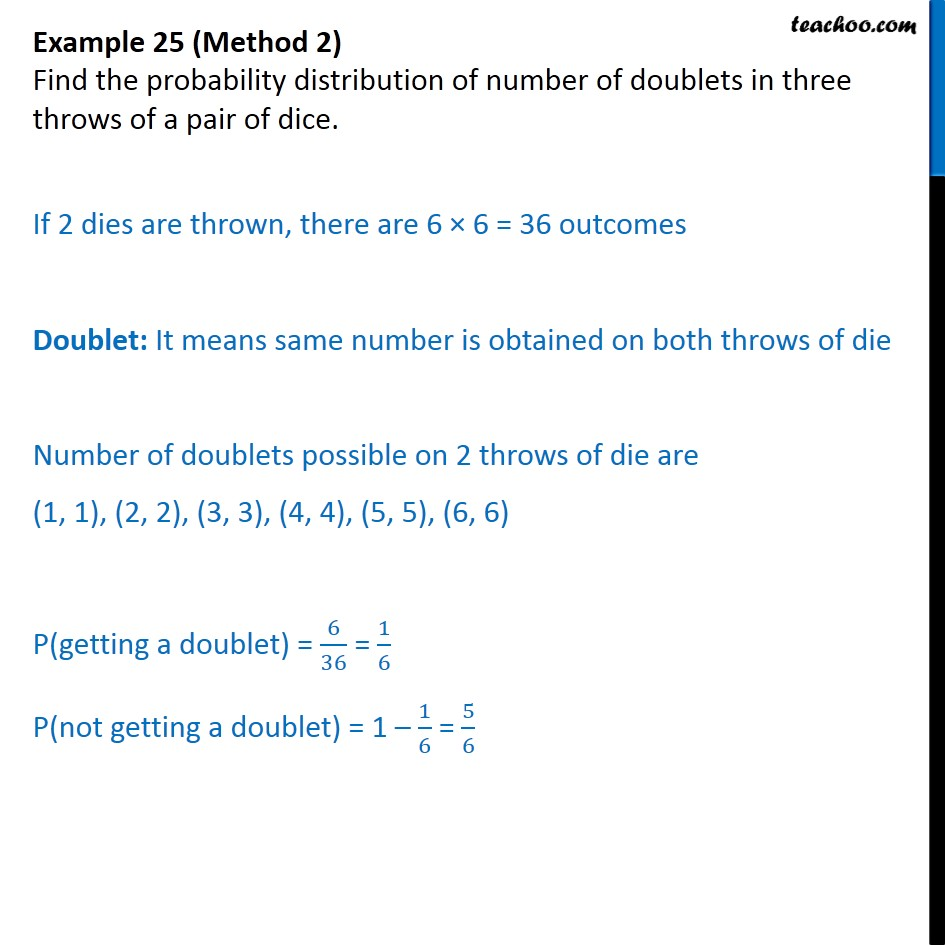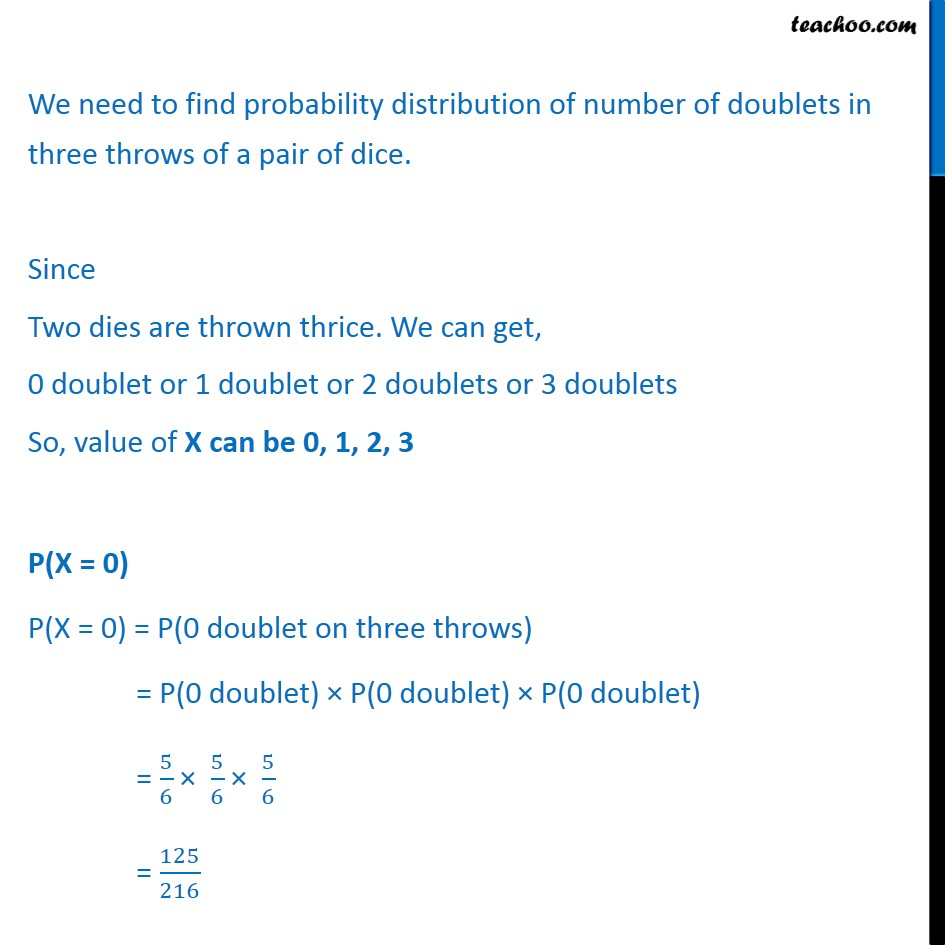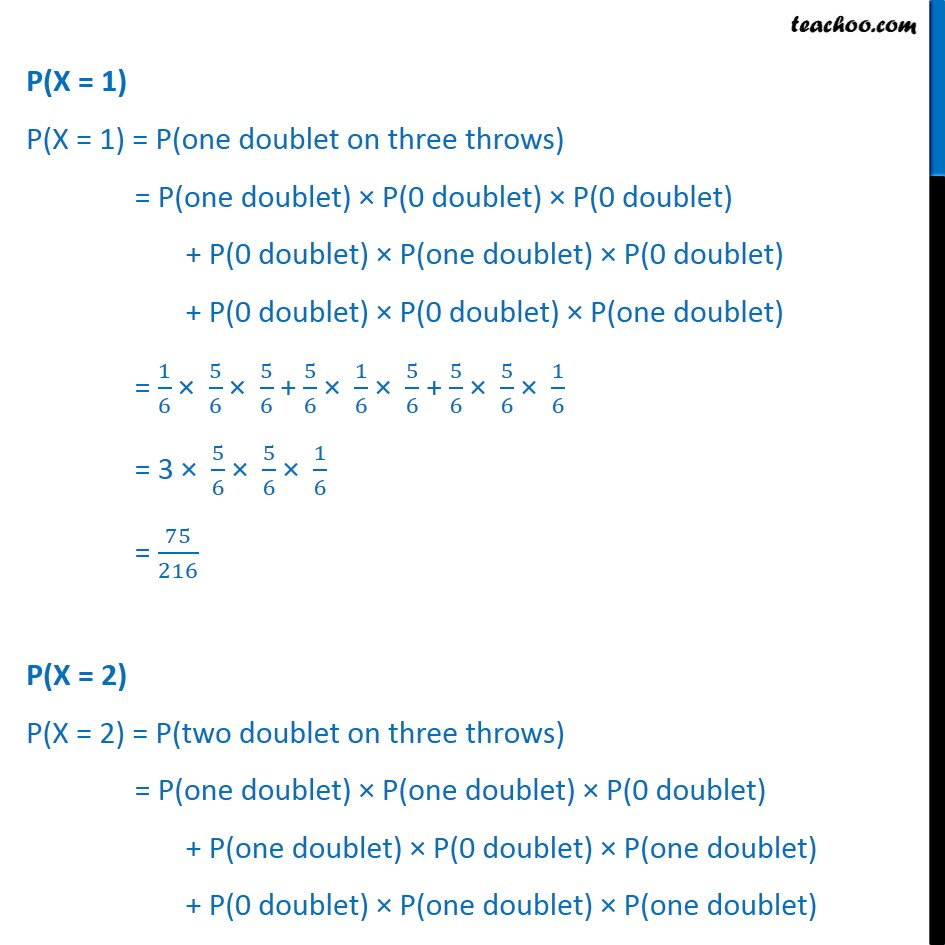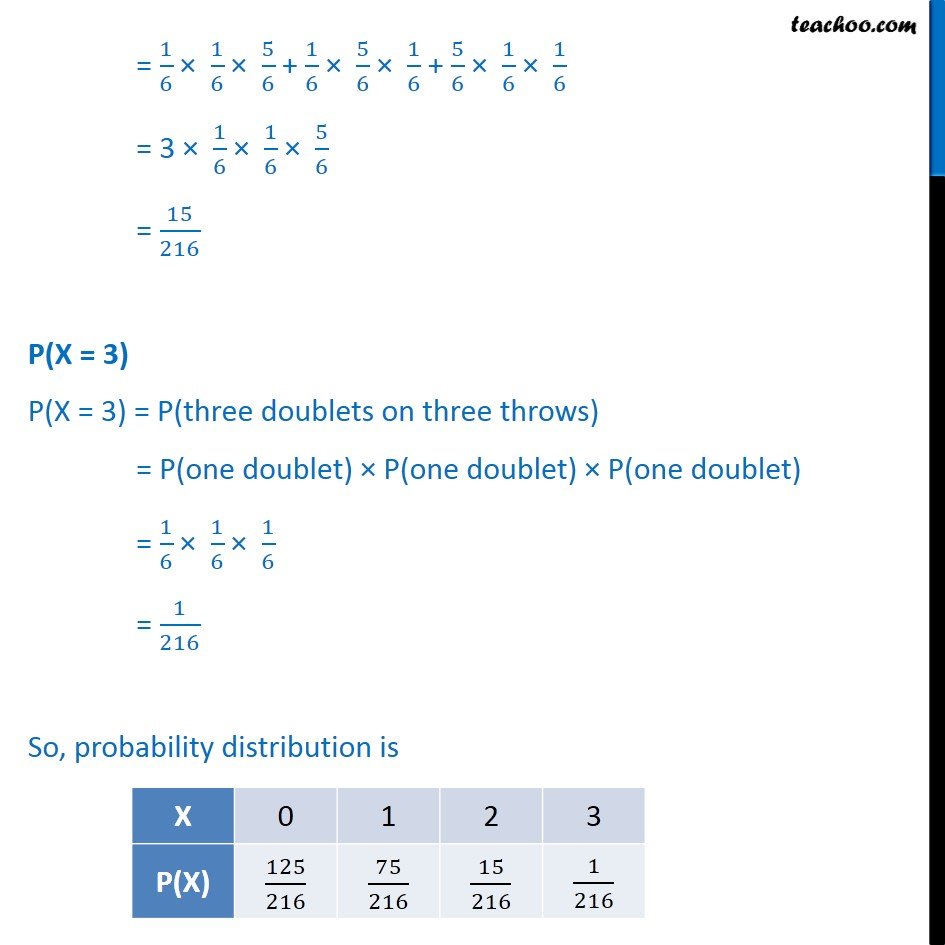1. Chapter 13 Class 12 Probability
2. Serial order wise
3. Examples

Transcript

Example 25 (Method 1) Find the probability distribution of number of doublets in three throws of a pair of dice. If 2 dies are thrown, there are 6 × 6 = 36 outcomes Doublet: It means same number is obtained on both throws of die Number of doublets possible on 2 throws of die are (1, 1), (2, 2), (3, 3), (4, 4), (5, 5), (6, 6) P(getting a doublet) = 6﷮36﷯ = 1﷮6﷯ P(not getting a doublet) = 1 – 1﷮6﷯ = 5﷮6﷯ We need to find probability distribution of number of doublets in three throws of a pair of dice. Let X : Number of doublets Throwing a die is a Bernoulli trial So, X has a binomial distribution P(X = x) = nCx 𝒒﷮𝒏−𝒙﷯ 𝒑﷮𝒙﷯ n = number of times pair of die is thrown = 3 p = Probability of getting doublet = 1﷮6﷯ q = 1 – p = 1 – 1﷮6﷯ = 5﷮6﷯ Hence, P(X = x) = 3Cx 𝟏﷮𝟔﷯﷯﷮𝒙﷯ 𝟓﷮𝟔﷯﷯﷮𝟑 − 𝒙﷯ Since Two dies are thrown thrice. We can get, 0 doublet or 1 doublet or 2 doublets or 3 doublets So, value of X can be 0, 1, 2, 3 Now, P(X = 0) = 3C0 1﷮6﷯﷯﷮0﷯ 5﷮6﷯﷯﷮3−0﷯= 1 × 1 × 5﷮6﷯﷯﷮3﷯= 125﷮216﷯ P(X = 1) = 3C1 1﷮6﷯﷯﷮1﷯ 5﷮6﷯﷯﷮3−1﷯= 3 × 1﷮6﷯ × 5﷮6﷯﷯﷮2﷯= 75﷮216﷯ P(X = 2) = 3C2 1﷮6﷯﷯﷮2﷯ 5﷮6﷯﷯﷮3−2﷯= 3 × 1﷮6﷯﷯﷮2﷯× 5﷮6﷯ = 5﷮216﷯ P(X = 3) = 3C3 1﷮6﷯﷯﷮3﷯ 5﷮6﷯﷯﷮0﷯= 1 × 1﷮6﷯﷯﷮3﷯ × 1 = 1﷮216﷯ So, probability distribution is Example 25 (Method 2) Find the probability distribution of number of doublets in three throws of a pair of dice. If 2 dies are thrown, there are 6 × 6 = 36 outcomes Doublet: It means same number is obtained on both throws of die Number of doublets possible on 2 throws of die are (1, 1), (2, 2), (3, 3), (4, 4), (5, 5), (6, 6) P(getting a doublet) = 6﷮36﷯ = 1﷮6﷯ P(not getting a doublet) = 1 – 1﷮6﷯ = 5﷮6﷯ We need to find probability distribution of number of doublets in three throws of a pair of dice. Since Two dies are thrown thrice. We can get, 0 doublet or 1 doublet or 2 doublets or 3 doublets So, value of X can be 0, 1, 2, 3 P(X = 0) P(X = 0) = P(0 doublet on three throws) = P(0 doublet) × P(0 doublet) × P(0 doublet) = 5﷮6﷯ × 5﷮6﷯ × 5﷮6﷯ = 125﷮216﷯ P(X = 1) P(X = 1) = P(one doublet on three throws) = P(one doublet) × P(0 doublet) × P(0 doublet) + P(0 doublet) × P(one doublet) × P(0 doublet) + P(0 doublet) × P(0 doublet) × P(one doublet) = 1﷮6﷯ × 5﷮6﷯ × 5﷮6﷯ + 5﷮6﷯ × 1﷮6﷯ × 5﷮6﷯ + 5﷮6﷯ × 5﷮6﷯ × 1﷮6﷯ = 3 × 5﷮6﷯ × 5﷮6﷯ × 1﷮6﷯ = 75﷮216﷯ P(X = 2) P(X = 2) = P(two doublet on three throws) = P(one doublet) × P(one doublet) × P(0 doublet) + P(one doublet) × P(0 doublet) × P(one doublet) + P(0 doublet) × P(one doublet) × P(one doublet) = 1﷮6﷯ × 1﷮6﷯ × 5﷮6﷯ + 1﷮6﷯ × 5﷮6﷯ × 1﷮6﷯ + 5﷮6﷯ × 1﷮6﷯ × 1﷮6﷯ = 3 × 1﷮6﷯ × 1﷮6﷯ × 5﷮6﷯ = 15﷮216﷯ P(X = 3) P(X = 3) = P(three doublets on three throws) = P(one doublet) × P(one doublet) × P(one doublet) = 1﷮6﷯ × 1﷮6﷯ × 1﷮6﷯ = 1﷮216﷯ So, probability distribution is

Examples